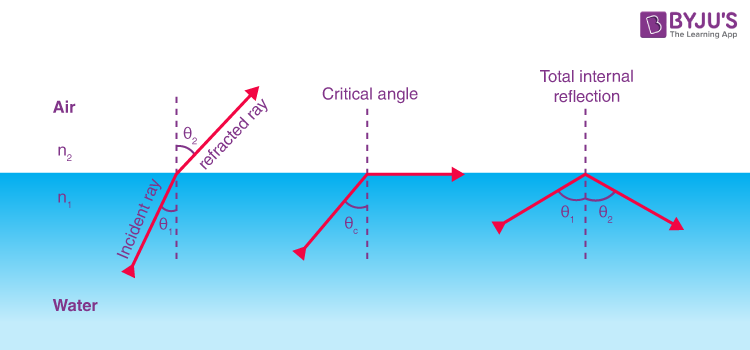# Total Internal Reflection

We know that light is the form of energy that can undergo various phenomena like reflection, refraction, dispersion, and total internal reflection. It is the phenomenon responsible for optical illusions like a mirage and also the reason for diamonds to shine. In this session, let us know more about the total internal reflection.

## What is Total Internal Reflection?

Total internal reflection is defined as:

The phenomenon which occurs when the light rays travel from a more optically denser medium to a less optically denser medium.Consider the following situation. A ray of light passes from a medium of water to that of air. Light ray will be refracted at the junction separating the two media. Since it passes from a medium of a higher refractive index to that having a lower refractive index, the refracted light ray bends away from the normal. At a specific angle of incidence, the incident ray of light is refracted in such a way that it passes along the surface of the water. This particular angle of incidence is called the critical angle. Here the angle of refraction is 90 degrees. When the angle of incidence is greater than the critical angle, the incident ray is reflected back to the medium. We call this phenomenon total internal reflection.## Formula of Total Internal Reflection

 Total internal reflection $$\begin{array}{l}\frac{n_{1}}{n_{2}}=\frac{sin r}{sin i}\end{array}$$ Critical angle, θ $$\begin{array}{l}sin \theta =\frac{n_{2}}{n_{1}}\end{array}$$

Notations Used In The Total Internal Reflection Formula And Critical Angle

• r is the angle of refraction
• i is the angle of incidence
• n1 is the refractive index in medium 1
• n2 is the refractive index in medium 2
• θ is the critical angle

## What are the Conditions of Total Internal Reflection?

Following are the two conditions of total internal reflection:

• The light ray moves from a more dense medium to a less dense medium.
• The angle of incidence must be greater than the critical angle.

Related Articles:

## Examples of Total Internal Reflection

Following are the examples of total internal reflection:

### Diamond:

When the incident ray falls on every face of the diamond such that the angle formed, the ray is greater than the critical angle. The critical value of the diamond is 23°. This condition is responsible for the total internal reflection in a diamond which makes it shine.

### Mirage:

It is an optical illusion that is responsible for the appearance of the water layer at short distances in a desert or on the road. Mirage is an example of total internal reflection which occurs due to atmospheric refraction.### Optical Fibre:

When the incident ray falls on the cladding, it suffers total internal reflection as the angle formed by the ray is greater than the critical angle. Optical fibres have revolutionised the speed with which signals are transferred, not only across cities but across countries and continents making telecommunication one of the fastest modes of information transfer. Optical fibres are also used in endoscopy.

## Solved Examples on Total Internal Reflection

Q1. An optical fibre made up the glass with refractive index n1 = 1.5 which is surrounded by another glass of refractive index n2. Find the refractive index n2 of the cladding such that the critical angle between the two cladding is 80°.

Solution:

Critical angle, θ = 80°

Refractive index, n1 = 1.5

Refractive index n= ?

Using the below formula, we can calculate n2:

$$\begin{array}{l}sin\theta =\frac{n_{2}}{n_{1}}\end{array}$$
$$\begin{array}{l}sin\;80^{\circ}=\frac{n_{2}}{1.5}\end{array}$$
$$\begin{array}{l}n_{2}=1.5\times sin80^{\circ}\end{array}$$
$$\begin{array}{l}n_{2}=1.48\end{array}$$

Q2. Find the refractive index of the medium whose critical angle is 40°.

Solution:

Critical angle, θ = 40°

Refractive index of the medium, μ = ?

$$\begin{array}{l}\mu =\frac{1}{sin\;\theta }\end{array}$$
$$\begin{array}{l}\mu =\frac{1}{sin\;40^{\circ} }\end{array}$$
$$\begin{array}{l}\mu =\frac{1}{0.65}\end{array}$$

µ = 1.6

Stay tuned with “BYJU’S – The learning app” to know more.

## Frequently Asked Questions – FAQs

Q1

### What is mirage?

Mirage is an optical illusion that is responsible for the emergence of the water layer at short distances in a desert or on the road.
Q2

### What is the formula to find the critical angle?

The formula to find the critical angle is:

$$\begin{array}{l}sin \theta =\frac{n_{2}}{n_{1}}\end{array}$$
Where,
n1 is the refractive index in medium 1
n2 is the refractive index in medium 2
Ө is the critical angle

Q3

### What is the formula to find the total internal reflection?

Total internal reflection is given by the formula:

$$\begin{array}{l}\frac{n_{1}}{n_{2}}=\frac{sin r}{sin i}\end{array}$$
Where,
r is the angle of refraction
i is the angle of incidence
n1 is the refractive index in medium 1
n2 is the refractive index in medium 2

Q4

True.
Q5

### Give some examples where optical fibre is used?

• Optical fibres are used in telecommunication for information transfer.
• It is also used in endoscopy.
• Stay tuned with BYJU’S to learn more interesting science topics with engaging videos!

Test your Knowledge on Total Internal Reflection